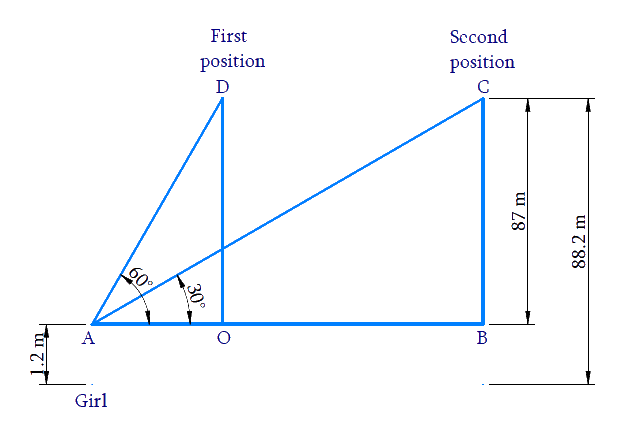# Ex.9.1 Q14 Some Applications of Trigonometry Solution - NCERT Maths Class 10

Go back to  'Ex.9.1'

## Question

A $$1.2 \,\rm{m}$$ tall girl spots a balloon moving with the wind in a horizontal line at a height of $$88.2\,\rm{m}$$  from the ground. The angle of elevation of the balloon from the eyes of the girl at any instant is $$60^\circ.$$ After some time, the angle of elevation reduces to $$30^\circ$$ see Figure. Find the distance travelled by the balloon during the interval.Video Solution
Some Applications Of Trigonometry
Ex 9.1 | Question 14

## Text Solution

What is Known?

(i) Height of the girl $$=$$ $$1.2\,\rm{m}$$

(ii) Vertical height of balloon from ground $$=$$ $$88.2\,\rm{m}$$

(iii) Angle of elevation of the balloon from the eyes of the girl is reducing from $$60^\circ$$ to $$30^\circ$$ as the balloon moves along wind.

(iv) From the figure, $$OD = BC$$ can be calculated as

$$88.2\,\rm{m}$$ $$–$$ $$1.2\,\rm{m}$$ $$=$$ $$87\,\rm{m}\dots$$  Result (1)

What is Unknown?

Distance traveled by the balloon, $$OB$$

Reasoning:

Trigonometric ratio involving $$AB,\, BC,\, OD,\,OA$$ and angles is tan$$\theta$$. [Refer $$AB,\, BC,\, OA$$ and $$OD$$ from the figure.]

Distance travelled by the balloon $$OB = AB − OA$$

Steps:

From the figure, $$OD = BC$$, can be calculated as

$$88.2\rm {m}\, – 1.2 \rm {m} = 87 {m}$$---------------- ($$1$$)

In $$\Delta AOD,$$

\begin{align}\tan {{60}^{0}}&=\frac{OD}{OA} \\ \sqrt{3}&=\frac{87}{OA} \\ OA&=\frac{87}{\sqrt{3}} \\ &=\frac{87}{\sqrt{3}}\times \frac{\sqrt{3}}{\sqrt{3}} \\ &=\frac{87\times \sqrt{3}}{3} \\& =29\sqrt{3}\text{m} \end{align}

In $$\Delta ABC,$$

\begin{align}\tan {{30}^{0}}&=\frac{BC}{AB} \\ \frac{1}{\sqrt{3}}&=\frac{87}{AB} \\ AB&=87\sqrt{3} \end{align}

Distance traveled by the balloon $$OB = AB \,– OA$$

\begin{align}OB & =87\sqrt{3}-29\sqrt{3} \\ & =58\sqrt{3} \end{align}

Distance traveled by the balloon $$= 58 \sqrt { 3 } \, \,\rm{m}$$

Learn from the best math teachers and top your exams

• Live one on one classroom and doubt clearing
• Practice worksheets in and after class for conceptual clarity
• Personalized curriculum to keep up with school